﻿ Characterization of Orthogonal Frequency Division Multiplexing (OFDM) Communication LinksPublications are Open
Access in this journal
Article Versions
Export Article
• Normal Style
• MLA Style
• APA Style
• Chicago Style
Communication
Open Access Peer-reviewed

Characterization of Orthogonal Frequency Division Multiplexing (OFDM) Communication Links

Rahmon Kamorudeen Adewale , Ogunti Erastus Olarewaju, Apena Waliu Olalekan
American Journal of Electrical and Electronic Engineering. 2020, 8(3), 91-97. DOI: 10.12691/ajeee-8-3-4
Received July 03, 2020; Revised August 04, 2020; Accepted August 13, 2020

Abstract

The demand for high data rate transmission is rapidly increasing in the area of wireless communications and Orthogonal Frequency Division Multiplexing (OFDM) is known to be a promising technique for high-rate transmission that can overcome the inter symbol interference (ISI) which results from the time dispersive nature of wireless channels. The idea is to utilize a number of carriers, spread regularly over a frequency band, in such a way so that the available bandwidth is utilized to maximal efficiency. This study presents the comparison of the performance of different modulation schemes: M-ary phase shift keying (M-PSK) and M-ary quadrature amplitude modulation (M-QAM) over Additive White Gaussian Noise (AWGN), Rayleigh and Rician multipath fading channels in OFDM system. Bit error rate (BER) was used to evaluate the performance of the system; the number of fast Fourier transform (FFT) points used during the transmission was also examined. The results showed that for all the channels, lower order modulation schemes perform better than the higher order schemes. This comes at the detriment of the data rate, as lower order schemes have lower data rates compared with their higher order counterparts. In addition, it was observed that the system performed better in AWGN channel than Rayleigh and Rician fading channels for all the modulation schemes used. It was observed that the number of FFT points used during the transmission do not have much effects on the performance of the system.

1. Introduction

The ever increasing usage of mobile phone technology, Wireless Local Area Network (WLAN) and the rapid growth of the mobile broadband industry have necessitated in finding new means of obtaining higher capacity wireless network 1. Wireless communication is the transfer of information between two or more points that are not connected by an electrical conductor, while wireless operations permit services such as; long-range communications, that are impossible or impractical to implement with the use of wires 2. Orthogonal frequency division multiplexing (OFDM) is a multi-carrier transmission technique that goes a long way in providing the much-needed improvement as it can be viewed as a multiplexing or modulation technique, which transmits at higher data rates and at the same time improves spectral efficiency 3.

OFDM utilises the spectrum more efficiently because the channels are much closer together unlike in frequency division multiple access (FDMA) where the channels are being spaced apart with a considerable distance to prevent inter-carrier interference and consequently reducing bit error rates. This eliminates the need for inter carrier guard bands used in FDMA thus simplifying the design of OFDM transceivers 4.

To prevent the inter-carrier interference in OFDM, the multiple carriers are made orthogonal to each other. Asides the higher data rate transmission and better spectral efficiency, OFDM offers other advantages like robustness against frequency-selective fading channel, efficient implementations using Fast Fourier Transform (FFT) and simple equalization techniques (low computational complexity) 5. These advantages have developed OFDM into a widely accepted strategy for wideband digital communication transmission used in areas such as digital television and audio broadcasting, wireless networks and 4th generation (4G) mobile communication 6. In accordance with the modulation order, the amount of error in the bit information can be varied 7. Thus, this study concentrated on the analysis of the performance of the OFDM system over different channels whilst employing different modulation techniques. The study was carried out using Simulink in MATLAB and the various digital modulation techniques. These modulation techniques include BPSK, QPSK, 16-QAM, 32-QAM and 64-QAM. The bit error rate (BER) performance of the OFDM system was evaluated over additive white Gaussian noise (AWGN), Rayleigh fading channels and Rician fading channel. The system was analysed over different FFT points to determine if the performance of the system is affected by this factor.

2. Principle of OFDM

A conventional OFDM symbol consists of multiple subcarriers, each carrying a single modulated symbol. An OFDM frame refers to a number of consecutive OFDM symbols 8. OFDM is a parallel transmission scheme, where a high-rate serial data stream is split up into a set of low rate substreams, each of which is modulated on separate subcarriers (SC). Thereby, the bandwidth of the SC becomes small compared with the coherence bandwidth of the channel; that is, the individual SC experience flat fading, which allows for simple equalization. This implies that the symbol period of the substreams is made long compared to the delay spread of the time dispersive radio channel. By selecting a special set of (orthogonal) carrier frequencies, high spectral efficiency is obtained because the spectra of the SC overlap, while mutual influence among the SC can be avoided 9. The bandwidth of the channel is orthogonally divided as shown in Figure 1.

• Figure 1. Orthogonal OFDM Spectrum Sub-Channels 
2.1. OFDM System Using FFT

A typical discrete-time baseband OFDM transceiver system is shown in Figure 2. The input data is processed by M- ary QAM or PSK modulation. The transmitter section converts digital data to be transmitted, into a mapping of subcarrier amplitude and phase. It then transforms this spectral representation of the data into the time domain using an Inverse Fast Fourier Transform (IFFT). These IFFT bins correspond to the orthogonal sub-carriers in the OFDM symbol. Therefore, the OFDM symbol can be expressed as equation (1) 10.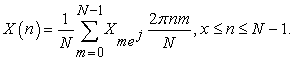(1)

Where X(n) is the modulated signal, N is the total numbers of subcarrier,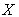is the amplitude of the subcarrier,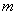is the subcarrier index and n is the time domain sample index of an OFDM signal.

After applying IFFT on the symbols, cyclic prefix is added and then passed through wireless communication channel. The digital data is converted to serial form and transmitted over the channel. At the receiver side, the process is reversed to obtain the data after the FFT, the signal is then converted back to the parallel form and demodulated to yield the transmitted signal back. The demodulated symbol stream is given by equation (2).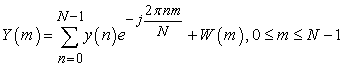(2)

Where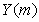is the demodulated signal, W(m) corresponds to the Additive White Gaussian Noise (AWGN) introduced in the channel.

• Figure 2. FFT Based OFDM System 
2.2. CP Insertion and Removal

Due to the multipath nature of the transmission of radio channel, inter-symbol interference (ISI) is introduced to the OFDM signals 11. Multipath transmission introduces ISI when the maximum delay spread of the radio channel is more than the guard interval length. Hence, the reason why the guard interval length is defined to be more than the maximum delay spread. Thus, the guard interval is added to mitigate the ISI effect 12. The CP is identical to the last samples of the OFDM symbols and is appended at the beginning of the symbol 13. Let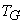denote the length of the cyclic prefix in terms of samples.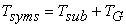(3)

Where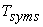is the total symbol duration,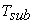is the useful symbol duration.

When the cyclic prefix is greater or equals to the maximum delay of a multipath channel, the ISI effect of an OFDM symbol on the next OFDM symbol is restricted to the guard interval so that the effective part of the next OFDM symbol would not be affected with the duration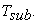• Figure 3. OFDM symbol with cyclic prefix
2.3. Orthogonality

Orthogonal Frequency Division Multiplexing (OFDM) is a multicarrier transmission technique, which divides the available spectrum into many carriers, each one being modulated by a low rate data stream. In Communications, multiple-access schemes are orthogonal when an ideal receiver can completely reject arbitrarily strong unwanted signals using different basis functions than the desired signal. Two signals are orthogonal to each other if they satisfy one condition. This condition requires the integral of two multiplied signals to be zero over a period of time. In fact, any two sinusoids are considered orthogonal provided that their frequencies are integer multiples of the same frequency. This is expressed in equation (4).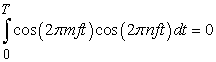(4)

Whereand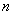indicate two integers, T is the period of the integration, which is the period of one symbol. It is convenient to set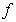to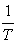in order to get the most efficiency from the OFDM system.

2.4. Digital Modulation in OFDM Systems

The nature of OFDM only allows the signals to be modulated in amplitude and phase only 5. This work focuses on PSK and QAM techniques only. The performance of these modulation techniques in a wireless communication system are evaluated in terms of bit error probability

For binary PSK (BPSK), which is the simplest form of PSK, a pair of signals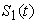and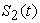which can be regarded as two phases separated by 180 degrees are used to represent two binary symbols; 1 and 0 respectively. For BPSK, the probability of bit error,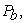is given 14 as equation (5).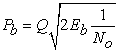(5)

Where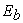is the Energy per bit,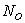is the noise power spectral density,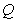is the error function frequently used for calculating the area under the tail of the Gaussian pdf denoted by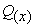in equation (6)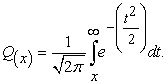(6)

For Quadrature PSK (QPSK), each symbol consists of 2 bits, that is k=2. Thus, four signals or message points are produced. These four points on the constellation diagram are equally spaced around a circle (usually spaced at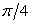). Hence, as QPSK’s symbol rate doubles that of BPSK, it is more spectral efficient than BPSK. The probability of bit error for QPSK is widely regarded as same as that of BPSK.

For Quadrature amplitude modulation (QAM), two digital bit streams are transmitted by modulating the amplitudes of the two carrier waves. The bit error probability,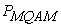for M-ary QAM scheme is given by: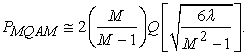(7)

For large value of M, equation (7) can be written as: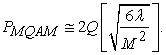(8)
2.5. Channels in OFDM System

In an OFDM system, the transmitter modulates the message bit sequence into PSK/QAM symbols, performs IFFT on the symbols to convert them into time-domain signals, and sends them out through a (wireless) channel. The received signal is usually distorted by the channel characteristics. In order to recover the transmitted bits, the channel effect must be estimated and compensated at the receiver 15.

2.5.1. Additive White Gaussian Noise Channel (AWGN)

Additive White Gaussian Noise is a basic noise model used to realise the effects of the many random processes that occur in a wireless channel. These random processes can come from some natural sources such as thermal noise and sun. The model does not account for fading, frequency selectivity, interference, non-linearity or dispersion 16. Instead, it provides simple models, which give a useful insight into the underlying behavior of a system before the aforementioned factors, are considered. Therefore, the model can be said to be useful in simulating the background noise of a channel 17. This model inputs a white Gaussian noise to the signal that passes through it for analysis purposes. For a transmitted signal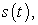the received signal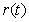is expressed as:(9)

Where,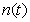is the additive white Gaussian noise.

The performance of the AWGN channel using the BPSK modulation technique can be evaluated using equation (10).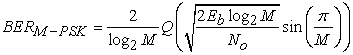(10)

Where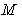is an integer and Q is given in equation (11).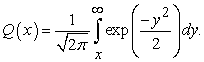(11)

2.5.2. Rayleigh Channel

In mobile radio channels, the Rayleigh distribution is commonly used to describe the statistical time varying nature of the received envelope of a flat fading signal, or the envelope of an individual multipath component. Rayleigh fading is a reasonable model when there are many objects in the environment that scatter the radio signal before it arrives at the receiver. The requirement that there be many scatters present means that Rayleigh fading can be a useful model in heavily built-up city centers where there is no line of sight between the transmitter and receiver and many buildings and other objects attenuate, reflect, refract and diffract the signal 18.

The received signal,in Rayleigh fading channel can be represented as 5.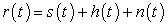(12)

Where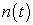is the AWGN with zero mean and unit variance,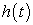is the random channel matrix having Rayleigh distribution,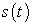transmitted signal.

The Rayleigh distribution is fundamentally the magnitude, z, of the sum of two equal independent orthogonal Gaussian random variables and would have a probability density function 5, 20.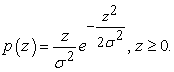(13)

The BER of the Rayleigh fading channel can be expressed as equation (14).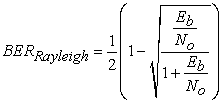(14)

Where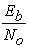is the signal-to-noise ratio.

2.5.3. Rician Channel

When there is a dominant stationary signal component present, such as a line-of-sight propagation path, the small scale fading envelope distribution is Rician. In such a situation, random multipath components arriving at different angles are superimposed on a stationary dominant signal. At the output of an envelope detector, this has the effect of adding a dc component to the random multipath. Rayleigh channel model is a stochastic model for radio propagation anomaly caused by partial cancellation of a radio signal by itself the signal arrives at the receiver by several different paths multipath interference and at least one of the paths is changing. Rician fading occurs when one of the paths, typically a line of sight signal, is much stronger than the others. In Rician fading, the amplitude gain is characterized by a Rician distribution 19.

3. Simulation Model

MATLAB toolbox was used to implement the methodology for this research. The performance results for the system using different modulation schemes were obtained using the OFDM parameters listed in Table 1.

3.1. OFDM Simulation Procedures

1. The system model was put together using the block diagram described in Figure 2, using MATLAB codes from the tool box.

2. The PSK and QAM modulation/demodulation codes were put in place whilst implementing only the AWGN channel.

3. The BER was observed from the BER calculator block

4. Steps 2 and 3 were repeated for the following modulation/demodulation block pairs: QPSK, BPSK, 16 QAM, 32 QAM, and 64QAM.

5. Steps 2, 3 and 4 were repeated while varying the number of subcarriers through the values: 256, 512 and 1024.

6. Steps 2, 3, 4 and 5 were repeated, using the AWGN alongside Rayleigh Fading channels

7. Steps 2, 3, 4 and 5 were repeated, using the AWGN alongside Rician Fading channels

4. Results and Discussion

The BER performances of different modulation techniques over AWGN channel in the OFDM configuration are presented.

The results are presented graphically which shows the BER as a function of the Eb/No. The modulation techniques considered are BPSK, QPSK, 16QAM, 32QAM and 64QAM. The BER against Eb/No for OFDM with different sub-carriers scenarios under AWGN channel are as shown in Figure 4 - Figure 6.

• Figure 4. BER for OFDM 256 subcarriers under AWGN channel
• Figure 5. BER for OFDM 512 subcarriers under AWGN channel
• Figure 6. BER for OFDM 1024 subcarriers under AWGN channel
4.1. BER Performance over AWGN Channel

It is seen that the system performs better as the Eb/No increases across all modulation techniques over the AWGN channel. It can also be seen that increasing the number subcarriers (FFT points) does not really affect the performance as they showed similar patterns. For example, 64QAM achieved an Eb/No of 8dB at BER of 0.0055 over AWGN when FFT point of 256, 512 and 1024 were employed. It is also observed that the lower order modulation techniques perform better than the higher order counterparts. BPSK and QPSK have similar performance while 16QAM performs better than both 32 QAM and 64 QAM. At FFT point of 512, 16 QAM achieves a BER of 0.01 at an Eb/No of around 5dB while it takes 32QAM 6dB and 64 QAM around 7dB to achieve the same level of BER. Similar patterns are obtained at other FFT points whilst employing a 25% of the FFT points as cyclic prefix in each case.

4.2. BER Performance over Rayleigh Channel
• Figure 7. BER for OFDM 256 subcarriers under Rayleigh channel
• Figure 8. BER for OFDM 512 subcarriers under Rayleigh channel
• Figure 9. BER for OFDM 1024 subcarriers under Rayleigh channel

From Figure 7 - Figure 9, it is seen that the performance has a similar pattern with AWGN only that the better BER are achieved at higher Eb/No. It is very clear that the system over AWGN outperforms the system over Rayleigh. It was observed that, lower order modulation still perform better than higher order modulation techniques. 16QAM gives a clearly better performance than 32QAM and 64 QAM. The numbers of subcarriers does not really have much effect on the system, BER of 0.001 achieved Eb/No of 8dB in 16QAM for all the FFT points considered. It can be deduced that as the Doppler frequency increases the BER is highly effected and it reduces the system performance in Rayleigh channel.

4.3. BER Performance over Rician Channel
• Figure10. BER for OFDM 256 subcarriers under Rician channel

From Figure 10 - Figure 12, it is seen that the performance has a similar pattern with AWGN and Rayleigh only that the better BER are achieved at higher Eb/No when compared with AWGN but at lower Eb/No when compared with Rayleigh. It is observed that lower order modulation still perform better than higher order modulation techniques. 16QAM gives a clearly better performance than 32QAM and 64 QAM. BPSK and QPSK perform better in all the channels. Generally, it can be concluded that increasing the number of FFT points in the OFDM system does not really have a significant effect on the performance of the system as no modulation technique showed a better performance over another. However, this could invariably affect the energy per bit of the signal.

• Figure 11. BER for OFDM 512 subcarriers under Rician channel
• Figure 12. BER for OFDM 1024 subcarriers under Rician channel

5. Conclusion

The BER performance of the FFT based OFDM system can be found over AWGN, RAYLEIGH and RICIAN fading channels using the M-QAM and M-PSK modulation schemes. From the performance of all the channels, it was found that BPSK modulation is better than other modulation schemes because it is more bandwidth efficient. In addition, the study investigated whether the number of FFT points employed has an effect on the system performance. The system performance was compared for all the employed modulation techniques, over all the channels. Generally, it can be concluded that over all the channels, lower order modulation schemes perform better than the higher order schemes but this comes at the expense of the data rate, as lower order schemes have lower data rates than their higher order counterparts. In addition, all the modulation schemes performs better in AWGN channel followed by RICIAN channel, RAYLEIGH has the least performance. When transmitting at higher subcarriers, no improvement is observed in any of the modulation schemes. Therefore, transmitting at high subcarriers generally does not have an effect on OFDM transmission although, transmit power could be affected. This performance evaluation can be adopted in Nakagami fading channel to evaluate its BER and compare it with other channels considered in this paper.

ReferencesThis work is licensed under a Creative Commons Attribution 4.0 International License. To view a copy of this license, visit http://creativecommons.org/licenses/by/4.0/